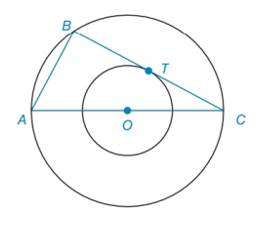Chapter 6.2, Problem 48EElementary Geometry For College St...

7th Edition
Alexander + 2 others
ISBN: 9781337614085

Solutions

Chapter
SectionElementary Geometry For College St...

7th Edition
Alexander + 2 others
ISBN: 9781337614085
Textbook Problem

Given concentric circles with center O , Δ A B C is inscribed in the larger circle, as shown. If B C ¯ is tangent to the smaller circle at point T and A B = 8 , find the length of the radius of the smaller circle.To determine

To find:

The length of the radius of the smaller circle.

Explanation

Calculation:

Given, concentric circles with center O.

ΔABC is inscribed in the larger circle as shown below.

It is also given that, BC¯ is tangent to the smaller circle at point T and AB=8.

Now draw a line joining O and T.

OT is the radius of smaller circle and OA is the radius of the bigger circle.

So, OTCT.

OTC=90°.

From the figure, ABBC.

So, ABC=90°

Therefore,

OTC=ABC

And again from the figure,

OCT=ACB

Hence, ΔOTCΔABC by AA similarity

Still sussing out bartleby?

Check out a sample textbook solution.

See a sample solution

The Solution to Your Study Problems

Bartleby provides explanations to thousands of textbook problems written by our experts, many with advanced degrees!

Get Started

Evaluate the expression sin Exercises 116. (2)3

Finite Mathematics and Applied Calculus (MindTap Course List)

Find the domain of the function. a. f(x) = 9x b. f(x) = x+32x2x3

Applied Calculus for the Managerial, Life, and Social Sciences: A Brief Approach

Evaluate the definite integral. 01ez+1ez+zdz

Single Variable Calculus: Early Transcendentals

Rewritten in reverse order,

Study Guide for Stewart's Multivariable Calculus, 8th

The general solution to (for x, y > 0) is: a) y = ln x + C b) c) y = ln(ln x + C) d)

Study Guide for Stewart's Single Variable Calculus: Early Transcendentals, 8th